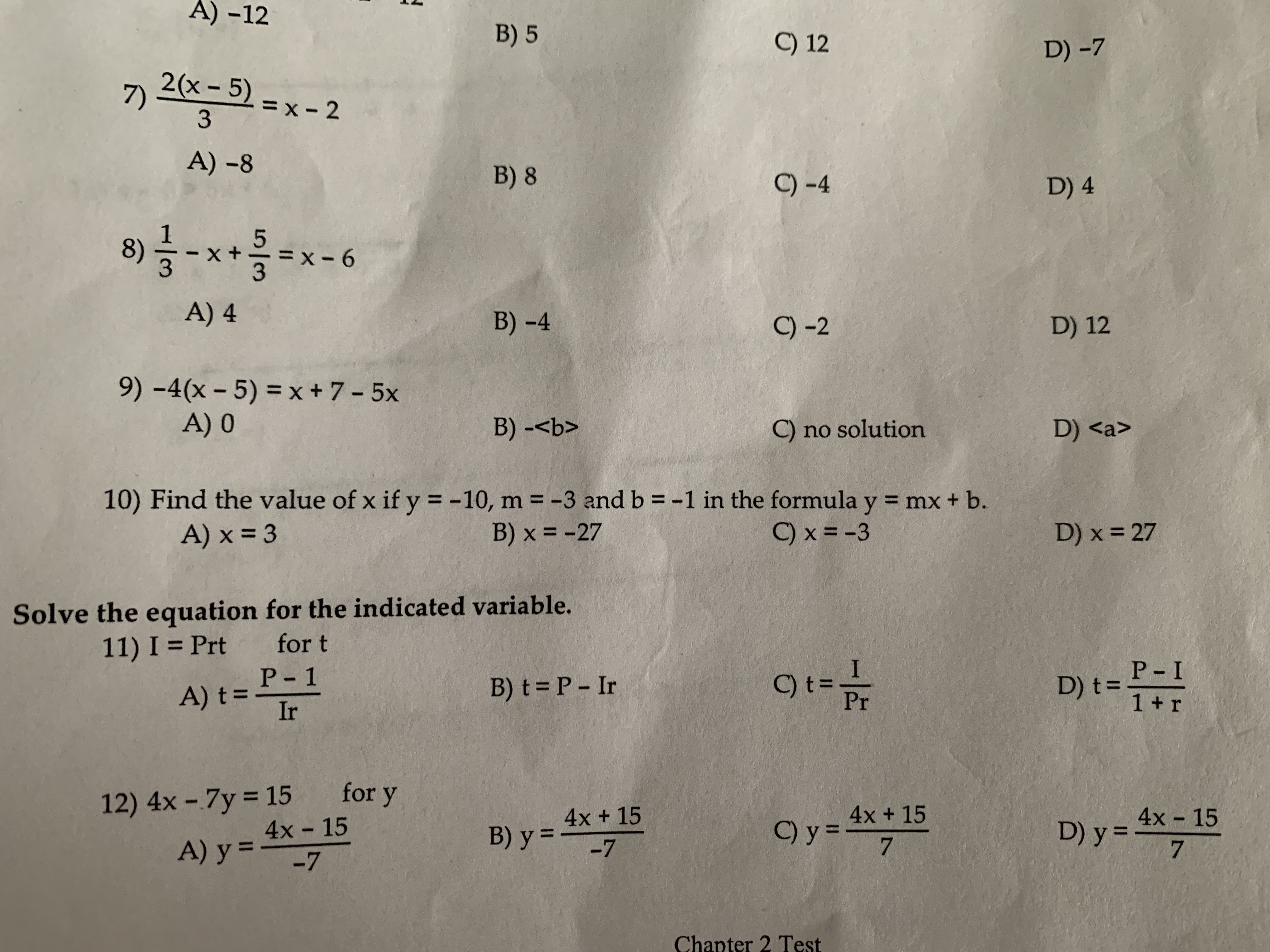# A)-12B) 5C) 12D) -72(x-5)7)=3A)-8B) 8C) -4D) 438) x+x-6A) 4B) -4C) -2D) 129)-4(x-5)=x+7-5xA) 0C) no solutionD) 10) Find the value of x if y =-10, m--3 and b--1 in the formula y = mx + b.B) x =-27A)x=3C)x=-3D) x = 27Solve the equation for the indicated variable.for tP-111) I = PrtC)t=PrP-I1 + rB) t=P-IrD) t-12) 4x-7y = 15for y4x 154x 154x +15D)y=4x-15Chapter 2 Test

Question
3 views

Number 10, please do step by stephelp_outlineImage TranscriptioncloseA)-12 B) 5 C) 12 D) -7 2(x-5) 7) = 3 A)-8 B) 8 C) -4 D) 4 3 8) x+x-6 A) 4 B) -4 C) -2 D) 12 9)-4(x-5)=x+7-5x A) 0 C) no solution D) 10) Find the value of x if y =-10, m--3 and b--1 in the formula y = mx + b. B) x =-27 A)x=3 C)x=-3 D) x = 27 Solve the equation for the indicated variable. for t P-1 11) I = Prt C)t=Pr P-I 1 + r B) t=P-Ir D) t- 12) 4x-7y = 15 for y 4x 15 4x 15 4x +15 D)y=4x-15 Chapter 2 Test fullscreen
check_circle

Step 1

The equation of a straight line with slope m and y intercept b is in the form of y = mx+b.

Here given slope m = -3 and yintercept b = -1.  we need to find the value of x for y = -10

Step 2

Substituting m = -3 and b = -1 we get the equati...

### Want to see the full answer?

See Solution

#### Want to see this answer and more?

Solutions are written by subject experts who are available 24/7. Questions are typically answered within 1 hour.*

See Solution
*Response times may vary by subject and question.
Tagged in

### Algebra